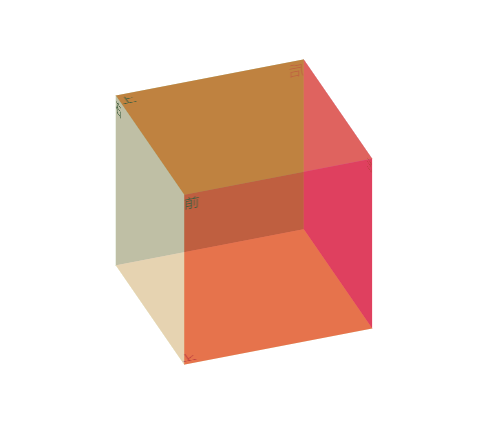• 随机生成或者手工输入两个立方体长宽高，打印出他们的颜色、表面积。 import random class Redcubic: color = 'red' def __init__(self, c, k, g): self.chang = c self.kuan = k self.gao = g self....
设计一个立方体的类Redcubic，包括三个实例属性：长、宽、高；一个类属性：立方体颜色；
随机生成或者手工输入两个立方体长宽高，打印出他们的颜色、表面积。
import random

class Redcubic:
color = 'red'

def __init__(self, c, k, g):
self.chang = c
self.kuan = k
self.gao = g
self.mianji = 2 * (c * k + c * g + k * g)

r1 = Redcubic(random.randint(1,10) , random.randint(1,10) , random.randint(1,10) )
r2 = Redcubic(random.randint(1,10) , random.randint(1,10) , random.randint(1,10) )

print(r1.chang, r1.kuan, r1.gao, r1.mianji, r1.color)
print(r2.chang, r2.kuan, r2.gao, r2.mianji, r2.color)

展开全文python random 大数据
• MFC在对话框的基础上实现根据用户指定的长宽高立方体
• 实验题目1：计算一个立方体盒子的体积 （1）程序功能简介 定义一个盒子（Box）类，数据成员包括长（length）、宽（width）、高（height）、体积（volume），能够使用构造函数对数据成员进行初始化，使用成员...
• 1.透视/景深效果 左手法则：大拇指指向当前坐标轴的下方向，手指环绕的方向就是正方向 perspective(length) 为一个元素设置三...比如你要建立一个立方体长宽高都是200px。如果你的perspective < 200px ，...
1.透视/景深效果

左手法则：大拇指指向当前坐标轴的下方向，手指环绕的方向就是正方向

perspective(length) 为一个元素设置三维透视的距离。仅作用于元素的后代，而不是其元素本身。当perspective:none/0;时，相当于没有设perspective(length)。比如你要建立一个小立方体，长宽高都是200px。如果你的perspective < 200px ，那就相当于站在盒子里面看的结果，如果perspective 非常大那就是站在非常远的地方看（立方体已经成了小正方形了），意味着perspective 属性指定了观察者与z=0平面的距离，使具有三维位置变换的元素产生透视效果perspective-origin属性规定了镜头在平面上的位置。默认是放在元素的中心transform-style：使被转换的子元素保留其 3D 转换(需要设置在父元素中)：
transform-style:flat 子元素将不保留其 3D 位置-平面方式。transform-style:preserve-3d 子元素将保留其 3D 位置—立体方式。
2.制作立方体html代码：
<body>
<div class="container">
<div class="front">前</div>
<div class="back">后</div>
<div class="left">左</div>
<div class="right">右</div>
<div class="top">上</div>
<div class="bottom">下</div>
</div>
</body>

css代码：
<style>
.container {
width: 200px;
height: 200px;
margin: 200px auto;
position: relative;
/* 使被转换的子元素保留其 3D 转换(需要设置在父元素中)： */
transform-style: preserve-3d;
/* 给立方体添加旋转动画 */
animation: rotate 20s linear infinite;
}

@keyframes rotate {
0% {
transform: rotateX(0deg) rotateY(0deg);
}

50% {
transform: rotateX(-10deg) rotateY(-360deg);
}

60% {
transform: rotateX(-10deg) rotateY(-340deg);
}

100% {
transform: rotateX(-360deg) rotateY(-340deg);
}
}

.container>div {
width: 200px;
height: 200px;
opacity: 0.5;
position: absolute;
left: 0;
top: 0;
}

.front {
background-color: red;
transform: translateZ(100px);
}

.back {
background-color: green;
transform: translateZ(-100px) rotateY(180deg);
}

.left {
background-color: purple;
transform: translateX(100px) rotateY(90deg);
}

.right {
background-color: pink;
transform: translateX(-100px) rotateY(-90deg);
}

.top {
background-color: orangered;
transform: translateY(-100px) rotateX(90deg);
}

.bottom {
background-color: yellowgreen;
transform: translateY(100px) rotateX(-90deg);
}
</style>

前端进阶精选：点此去
展开全文html5 css3
• 设计一个立方体的类，求出立方体的面积和体积 */ class Cube { public: //求出立方体的面积和体积 int cal_cube_S(int h,int w, int l) { int c_H = h; int c_W = w; int c_L = l; int c_S; c_S = c_H * ...
#include<iostream>
using namespace std;
/*
设计一个立方体的类，求出立方体的面积和体积
*/
class Cube
{
public:
//求出立方体的面积和体积
int cal_cube_S(int h,int  w, int l)
{
int c_H = h; int c_W = w; int c_L = l;
int c_S;
c_S = c_H * c_W * 2 + c_L * c_W * 2 + c_H * c_L * 2;
return c_S;
}
int cal_cube_V(int h, int  w, int l)
{
int c_H = h; int c_W = w; int c_L = l;
int c_S, c_V;
c_V = c_H * c_W * c_L;
return c_V;
}
void print(int h, int  w, int l, int S, int V)
{
cout << "长：" << l << "  宽：" << w << "  高：" << h << endl;
cout << "表面积：" << S << endl;
cout << "体积：" << V << endl;

}
private:
int c_H, c_W, c_L;
};
int main()
{
int l1=10, w1=10, h1=10;
int l2 = 10, w2 = 10, h2 = 10;
Cube cube;
int S1=cube.cal_cube_S(l1,w1,h1);
int V1 = cube.cal_cube_V(l1, w1, h1);
int S2 = cube.cal_cube_S(l2, w2, h2);
int V2=cube.cal_cube_V(100, 10, 10);
cube.print(h1, w1, l1, S1, V1);
cube.print(h2, w2, l2, S2, V2);
system("pause");
return 0;
}

展开全文• 设置并获取立方体类的长宽高 //2.获取立方体类的面积和体积 //3.实例化两个立方体 //4.运用全局函数和成员函数判断两个立方体是否相等 #include<iostream> #include<cmath> using namespace std; class...
题目:求出立方体类 设计立方体类 求出立方体的面积和体积 分别用全局函数和成员函数判断两个立方体是否相等
//1.设置并获取立方体类的长宽高
//2.获取立方体类的面积和体积
//3.实例化两个立方体
//4.运用全局函数和成员函数判断两个立方体是否相等
#include<iostream>
#include<cmath>
using namespace std;
class Cube{
private:
int m_L;
int m_w;
int m_H;
public:
void setL(int l)
{
m_H=l;
}
int getL()
{
return m_L;
}
void setW(int w)
{
int m_W=w;
}
int getW()
{
int m_W;
return  m_W;
}
void setH(int h)
{
m_H=h;
}
int getH()
{
return m_H;
}
int calculateS()
{
int m_W,m_L,m_H;
return 2*m_L*m_W+2*m_L*m_H+2*m_W*m_H;
}
int calculateV()
{
int m_W,m_L,m_H;
return m_L*m_W*m_H;
}
bool isSamebyclass(Cube &c)
{
int m_W;
if(m_L==c.getL()&&m_W==c.getW()&&m_H==c.getH())
{
return true;
}
return false;
}
};
bool isSame(Cube &c1,Cube &c2)
{
if(c1.getL()==c2.getL()&&c1.getW()==c2.getW()&&c1.getH()==c2.getH())
{
return true;
}
return false;
}
int main()
{
Cube c1;
c1.setL(2);
c1.setW(2);
c1.setH(2);
cout<<"立方体的面积为："<<c1.calculateS()<<endl;
cout<<"立方体的体积为："<<c1.calculateV()<<endl;
Cube c2;
c2.setL(2);
c2.setW(2);
c2.setH(2);
cout<<"立方体的面积为："<<c2.calculateS()<<endl;
cout<<"立方体的体积为："<<c2.calculateV()<<endl;
bool ret=isSame(c1,c2);
if(ret)
{
cout<<"c1和c2是相等的"<<endl;
}
else
{
cout<<"c1和c2是不相等的"<<endl;
}
ret=c1.isSamebyclass(c2);
{
if(ret)
{
cout<<"c1和c2是相等的"<<endl;
}
cout<<"c1和c2是不相等的"<<endl;
}
}

复盘到的东西: 1.成员属性设为私有，可以自己控制读写权限，需要时设置接口输出即可，因此在定义类的属性时，首选为私有。
2.判断两个立方体是否相等，也就是要判断他们的长宽高是否相等，在判断时，选用bool函数
3.全局函数在类外定义即可，而成员函数作为类的一份子在类内定义
4.成员函数由类内的一个立方体调动，故在传值时只需传进一个立方体的值即可
展开全文• 已知空间上一点是立方体一个顶点（这里假设该点为最靠近坐标原点的点），和长宽高值。如何再python中画出改立方体？ matplotlib可以实现么？python
• word中怎么插入长方体正方体如何绘制如何在文档中画出正方体的三视图的方法1 在桌面上找到CAXA的启动程序，左键双击启动程序；...catia文件中怎么把一个长方形变成一个立方体可实现的方法很多: 1.关联参数,...
• 设计一个立方体类Box，它能计算并输出立方体的体积和表面积。其中Box类包含三个私有数据成员 a(立方体边长)volume(体积)和 area(表面积)，另有两个构造函数以及getvolume（）(计算体积)、getarea()(计算表面积)和 ...c++
• 3、分别用全局函数和成员函数判断两个立方体是否相等。 #include<iostream> #include<string> using namespace std; //创建立方体类 class Cube { private: //属性 一般设置为私有 长宽高 int m_L;...c++
• 设计一个立方体类Box，它能计算并输出立方体的体积和表面积,并编写main函数测试该类。 其中Box类包含三个私有数据成员a(立方体边长)、volume(体积)和area(表面积)，另有两个构造函数以及seta()(设置立方体边长)、...
• //创建立方体类并分别用全局函数和成员函数判断两个立方体是否相等 #include<iostream> using namespace std; //创建立方体类 class cube { public: //给立方体初始化 void set(int l,int w,int h) { m_L...c++
• 设置和获取立方体长宽高 //属性： //长 宽 高 //在全局函数和成员函数下分别判断两立方体是否相等 class cube { public: //行为： //设置长 void set_L(int l=0) { m_l = l; } //获取长 int get_L() { ...c++
• 问题：如何利用sandy的类库去创建一个立方体呢？ 首先，我们要先了解sandy类库的结构 可以看到上面就官方网放出的类库表，有一些很容易理解，像sandy.events 这个和flash.event 事件都差不多，如果我们...
• 一个from中绘制立方体，之后绘制小的立方体填充大的立方体
• css3实践—创建3D立方体 　要想实现3D的效果，其实非常简单，只需指定一个元素为容器并设置transform-style：preserve-3d，那么它的后代元素便会有3D效果。不过有很多需要注意的地方，这里把我学习的方法，过程...
• 一个立方体有六个面。所以，它的HTML结构应该是这样的(这里用Vue.js来实现)：拍岸的布落格为了便于理解，我们给每个面一个单独的class，好作区分，你也可以用cube__item:nth-of-type(1/2/3/4/5/6)这样的伪类来写。...
• 在C++环境下画立方体，有长宽高的设置 对话框来实现设置
• 使用面向过程的方法： #include <iostream>... //设置立方体长宽高 void setABC(int a, int b, int c){ m_a = a; m_b = b; m_c = c; } int getA() { return m_a; }
• 要想实现3D的效果，其实非常简单，只需指定一个元素为容器并设置transform-style：preserve-3d，那么它的后代元素便会有3D效果。不过有很多需要注意的地方，这里把我学习的方法，过程分享给大家。再讲知识点之前，...css3 3d
• 立方体贴图就是一个包含了6个2D纹理的纹理，每个2D纹理都组成了立方体一个面 他的一个优点是可以通过他的坐标直接当作他的纹理坐标（一个长宽高为1的正方体盒子，以原点为中心，他的坐标就是他的纹理坐标） 如果...
• 透视/景深效果 a) perspective(length)为...比如你要建立一个立方体长宽高都是200px，如果你的perspective&lt;200px,那就相当于站在盒子里面看的结果，如果perspective非常大，那就相当于站在非常远的地方看，...
• 计算立方体体积 #define _CRT_SECURE_NO_WARNINGS #include<...分别用全局函数和成员函数判断两个立方体是否相等。 */ class Cube { public: //设置长宽高 void setL(int l) { m_L = l; } void sec++
• class cube{ //创建立方体类。 public: void send_h(double a){ //输入高的成员函数。 h = a; } void send_w(double b){ //输入宽的成员函数。 w = b; } void send_l(double c){ //输入长的成员...c++
• （两个立方体相等当且仅当长宽高都相等。） 法：利用成员函数判断 #include<iostream> using namespace std; class Cube { public: void setLong(int length) { c_L = length; }//可写，设置长 int ...c++
• 立方体表面积，可以计算立方体以及抽壳后的体积及重量！非常方便。...